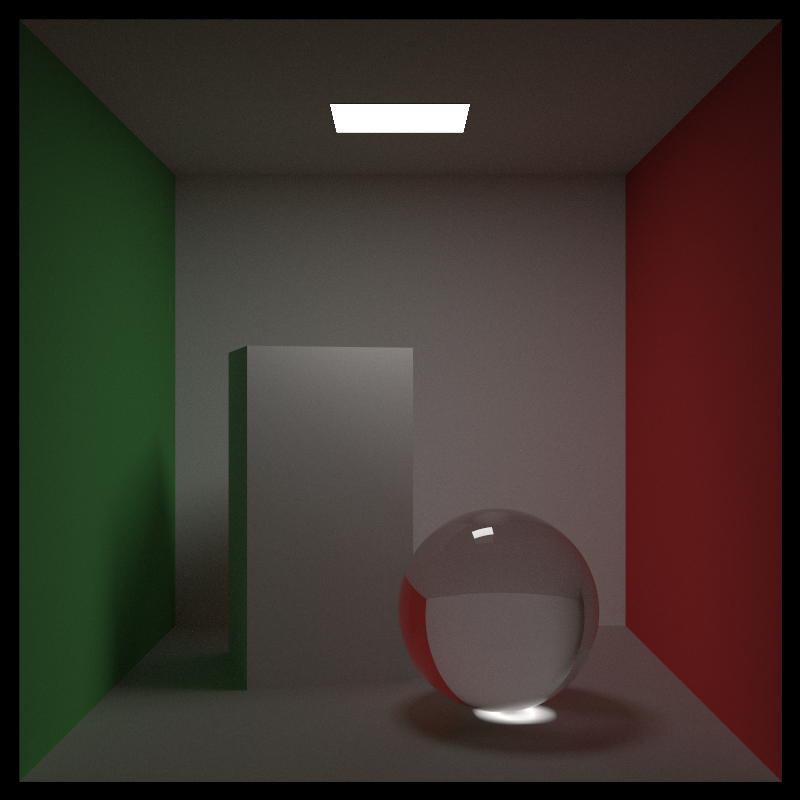# Crate ray_tracing_core

GitHub page rabbid76.github.io/ray-tracing-with-rust
GitHub repository Rabbid76/ray-tracing-with-rustBased on Peter Shirley’s books:“Note that I avoid most “modern features” of C++, but inheritance and operator overloading are too useful for ray tracers to pass on.”
Peter Shirley, Ray Tracing in One Weekend

# Example

```use ray_tracing_core::random;
use ray_tracing_core::test::TestSceneSimple;
use ray_tracing_core::types::ColorRGB;
use ray_tracing_core::types::FSize;

fn main() {
let cx = 40;
let cy = 20;
let samples = 10;
let scene = TestSceneSimple::new().scene;

let mut pixel_data: Vec<u8> = Vec::with_capacity(cx * cy * 4);
pixel_data.resize(cx * cy * 4, 0);

for y in 0..cy {
for x in 0..cx {
let mut c = ColorRGB::new(0.0, 0.0, 0.0);
for _ in 0..samples {
let u = (x as FSize + random::generate_size()) / cx as FSize;
let v = 1.0 - (y as FSize + random::generate_size()) / cy as FSize;
c = c + scene.ray_trace_color(u, v);
}
c = c / samples as FSize;

let i = (y * cx) + x;
pixel_data[i * 4] = (c.sqrt() * 255.0).round() as u8;
pixel_data[i * 4 + 1] = (c.sqrt() * 255.0).round() as u8;
pixel_data[i * 4 + 2] = (c.sqrt() * 255.0).round() as u8;
pixel_data[i * 4 + 3] = 255;
}
}

// [...]
}```

## Modules

 core Ray Trace core environment Environment hit_able Hit able objects material Material objects math Ray Trace Math probability_density_function Probability Density Function random Random data generator test Internal module for test for integration tests texture Texture objects types Ray tracing data types